Question

the Pythagorean theorem to find the length of the unknown side of a right triangle, where...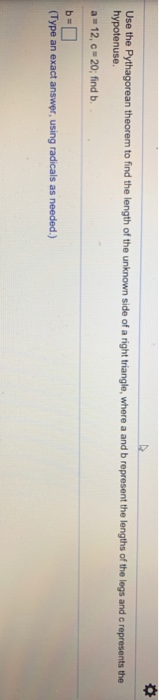the Pythagorean theorem to find the length of the unknown side of a right triangle, where a and b represent the lengths of the legs and c represents the hypotenuse. a 12, c 20, find b (Type an exact answer using radicals as needed.)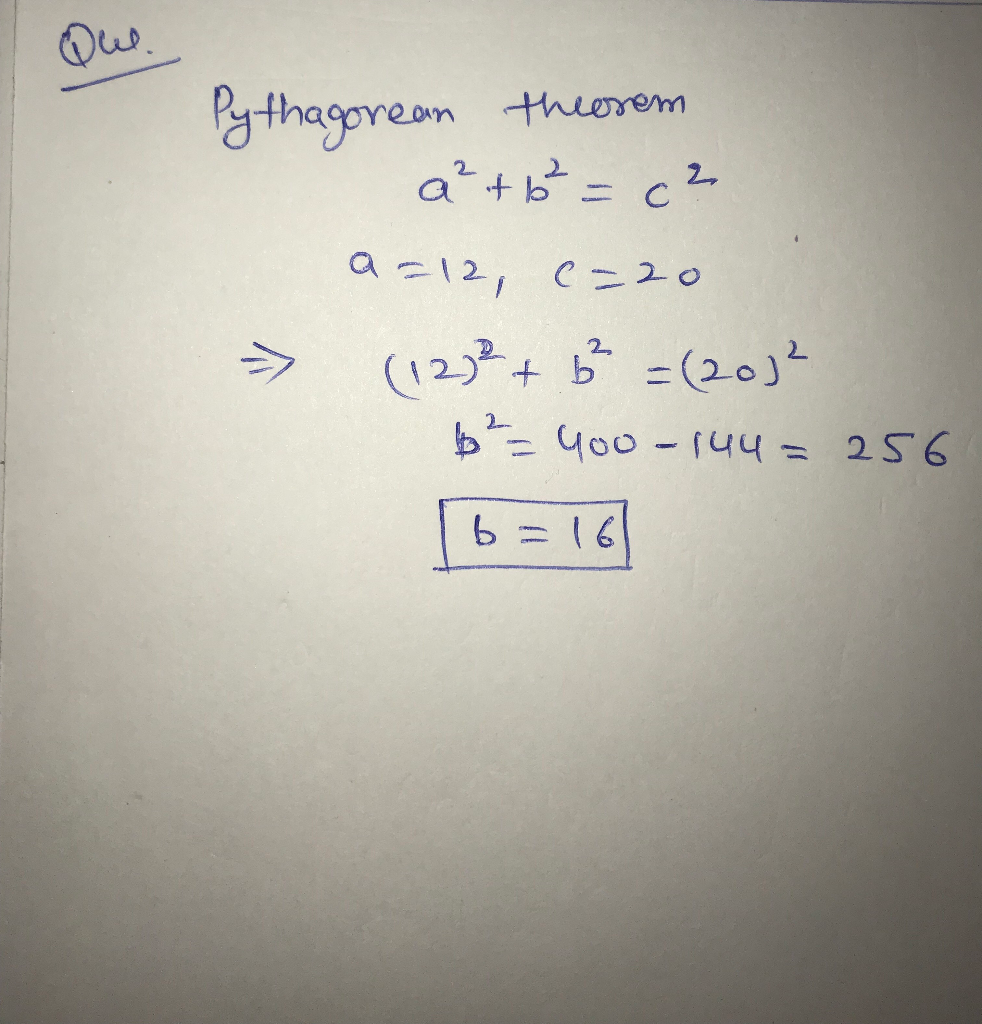Earn Coins

Coins can be redeemed for fabulous gifts.

Similar Homework Help Questions
• Let a and b be the lengths of the legs of a right triangle, and c...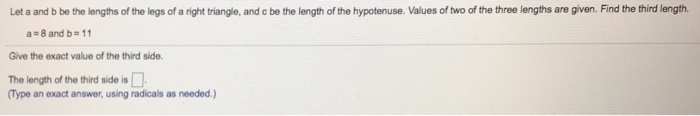Let a and b be the lengths of the legs of a right triangle, and c be the length of the hypotenuse. Values of two of the three lengths are given. Find the third length. a 8 and b 11 Give the exact value of the third side. The length of the third side is Type an exact answer, using radicals as needed.)

• The Pythagorean Theorem for right triangles states that if a and b are the lengths of the legs of a right triangle and c is the length of the hypotenuse, then a2 C b2 D c2

The Pythagorean Theorem for right triangles states that if a and b are thelengths of the legs of a right triangle and c is the length of the hypotenuse,then a2 C b2 D c2

• B Use the Pythagorean Theorem to find the length of the missing side of the right...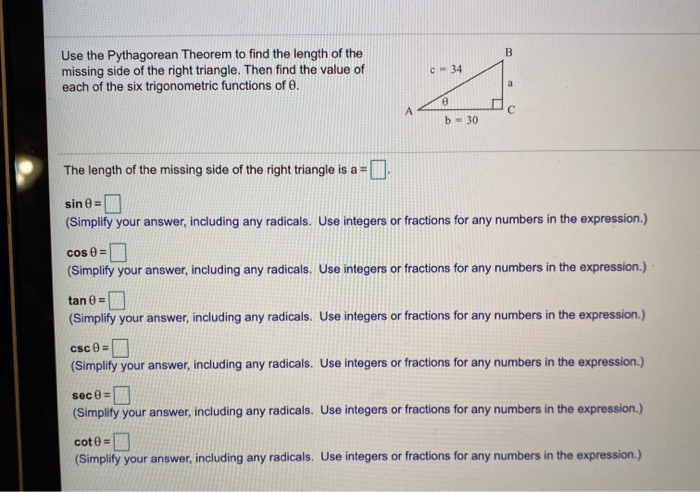B Use the Pythagorean Theorem to find the length of the missing side of the right triangle. Then find the value of each of the six trigonometric functions of e. C 34 a A C b-30 The length of the missing side of the right triangle is a = sin = 1 (Simplify your answer, including any radicals. Use integers or fractions for any numbers in the expression.) cos = (Simplify your answer, including any radicals. Use integers or fractions...

• Question 14 Use the Pythagorean Theorem to find the length of the missing side of the...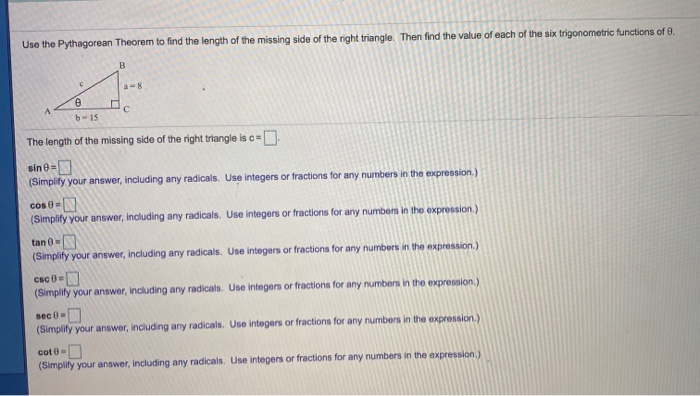Question 14 Use the Pythagorean Theorem to find the length of the missing side of the right triangle. Then find the value of each of the six trigonometric functions of 8. B A с b-15 The length of the missing side of the right triangle is c=0 sine- (Simplify your answer, including any radicals. Use integers or fractions for any numbers in the expression.) cos 0 = (Simplify your answer, including any radicals. Use integers or fractions for any numbers...

• Find the exact length of the unknown side of the triangle shown to the night. Do...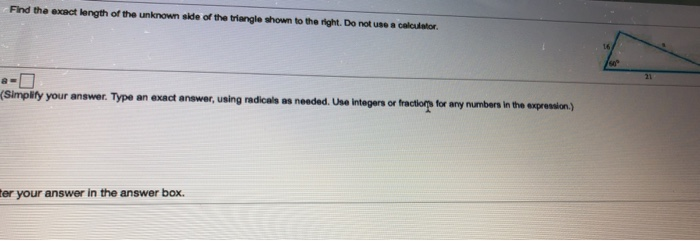Find the exact length of the unknown side of the triangle shown to the night. Do not use a cultor 16 60° 21 (Simplify your answer. Type an exact answer, using radicals as needed. Use integers or fractions for any numbers in the expression) ter your answer in the answer box.

• and and right angle at C. Find the unknown side length using the Pythagorean Reorem and...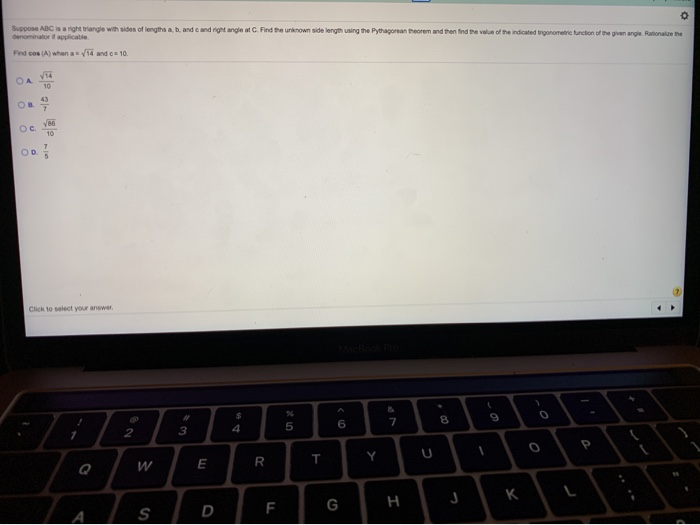and and right angle at C. Find the unknown side length using the Pythagorean Reorem and then find the value of the indicated trigonometric function of the genergie Rain the Suppo ABC is a night triangle with sides of length denomin a pplicable Find os (A) when a 14 and c= 10 Cac to you

• Use the Pythagorean Theorem to find the exact length of the missing side of each below....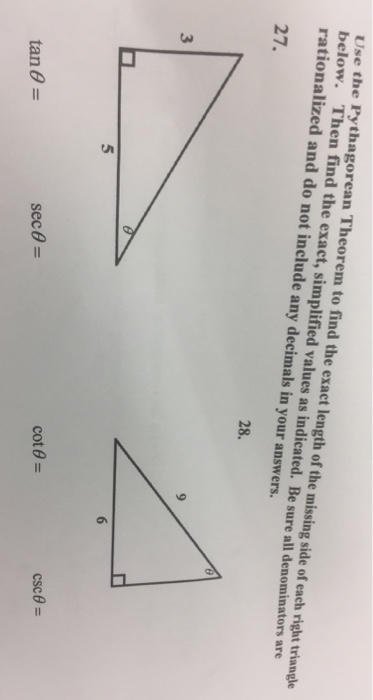Use the Pythagorean Theorem to find the exact length of the missing side of each below. Then find the exact, simplified values as indicated. Be sure all denominators are rationalized and do not include any decimals in your answers right triangle 27. 28. 3 5 cote-

• Without using the Pythagorean theorem, prove that two right triangles are congruent if the hypotenuse and...

Without using the Pythagorean theorem, prove that two right triangles are congruent if the hypotenuse and leg of one are equal to the hypotenuse and leg of the other.   Do this with placing the triangles so that there equal legs coincide and their right legs are adjacent. This will form a large isoceles triangle. Use this to show that the given triangles are congruent by AAS.

• Using Pythagorean's Theorem, find the hypotenuse if the two sides have a length of 300m and...

Using Pythagorean's Theorem, find the hypotenuse if the two sides have a length of 300m and 400m (Pythagorean's Theorem states that a^2 + b^2 = c^2, where a and b are the lengths of the sides of a right triangle and c is the length of the side opposite the 90 degree angle).

• 11) (10 points) Use the Pythagorean Theorem to find the length of the missing side of...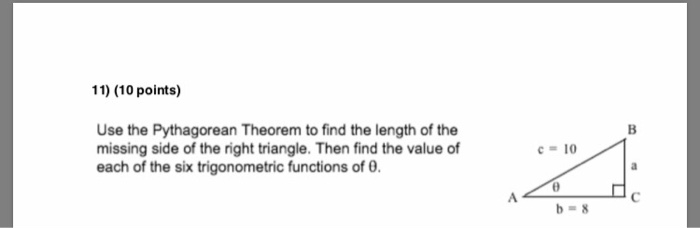11) (10 points) Use the Pythagorean Theorem to find the length of the missing side of the right triangle. Then find the value of each of the six trigonometric functions of 0